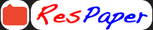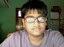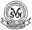Trending ▼   ResFinder# ICSE Class X Notes 2019 : Physics

3 pages, 4 questions, 0 questions with responses, 0 total responses,10Sital Mahapatra Vidyasagar Shishu Niketan (VSN), West Medinipur IX
+Fave Message
 Home > atri001 >   F Also featured on: rikhil32Formatting page ...

Formulae of Physics SN Formulae Remarks Force, Work, Power, Energy 1. 2. W=F S W=Fcos S 3. 1kJ=103 J 1MJ=106 J 1 J =107 erg 1 W h=3600 J 1 kW h=3.6 x 106 J 1 cal = 4.86 J 1 eV = 1.6x1019 J P = W/t 1 W = 1J/s =107 erg/s 1 kW = 103 W 1 MW = 106 W 1 GW = 109 W 1 KE= 2 m v2 PE = mgh L MA= E VR= VE / VL VR = dE / dL Wi = E x dE W o = L x dL =Po / Pi = Wo / Wi VR = MA x Load x Load Arm = Effort x Effort Arm Class II Lever = Force Multiplier Class III Lever = Speed Multiplier MA = VR = n (total number of pulley in Block & tackle system) MA = n w/E, =1-w/nE MA= 1/h = 1/sin Light c = v 4. 5. 6. 7. 8. 9. 10. 11. 12. 13. 14. 15. 16. 17. 18. 19. 20. 21. 22. 23. 24. 25. 26. V= v 1 2 = 2 / 1 =Vair or vacuum/Vmedium 1 2 = sin i / sin r In the direction of force In the direction of displacement SI to CGS Scalar quantityFormatting page ...Formatting page ...